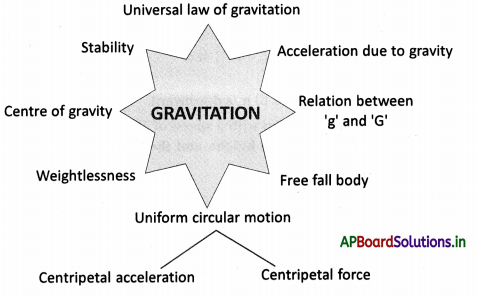# AP Board 9th Class Physical Science Notes Chapter 8 Gravitation

Students can go through AP State Board 9th Class Physical Science Notes Chapter 8 Gravitation to understand and remember the concept easily.

## AP State Board Syllabus 9th Class Physical Science Notes Chapter 8 Gravitation

→ Uniform circular motion is a motion of the body with a constant speed in a circular path

→ The acceleration which can change only the direction of the velocity of a body is called centripetal acceleration.

→ The net force which can change only the direction of the velocity of a body is called centripetal force directed towards the centre of the circle.

→ Centripetal force Fc = mv2/R

→ The universal law of gravitation states that everybody in the universe attracts other bodies within a force that is directly proportional to the product of their masses and inversely proportional to the square of the distance between them.

→ Universal gravitational constant G = 6.67 × 10-11 Nm2 kg-2

→ Acceleration due to gravity (g) is independent of the mass of the body

→ When a body is thrown up ‘g’ is taken as negative and for a freely falling body, ‘g’ is positive.

→ A body is said to be free-falling when it starts with ‘zero’ velocity and travelling vertically downward.→ During free-fall conditions, the body is in a state of weightlessness.

→ The point where total weight appears to act is called the ‘centre of gravity.

→ If we draw a line straight down from the centre of gravity of an object of any shape and it falls inside the base of the object, then the object will be stable

→ If the line through the centre of gravity falls outside the base then the object will be unstable.

→ The body is in equilibrium when the weight vector goes through the base of the body.

→ The distance of the moon from the centre of the earth is 384400 km (or) 3.844 × 1010 cm.

→ The time period of the moon around the earth = 27.3 days (or) 2.35 × 106 s.

→ Acceleration of bodies acquired near the surface of earth ‘g’ = 981 cm/s2 (or) 9.81 m/s2

→ Radius of earth Re = 6.4 × 106 km.

→ Universal gravitational constant G = 6.67 × 10-11 Nm2 kg-2.

→ Mass of the earth (M) = 6 × 1024 kg.→ Uniform circular motion: The motion of a body with constant speed in a circular path is called uniform circular motion.

→ Centripetal acceleration: The acceleration which causes changes only in the direction of the velocity of a body is called centripetal acceleration and it is always directed towards the centre of the circle.

→ Centripetal force: The net force required to keep a body in uniform circular motion is called centripetal force.
F = mv2/ R

→ Centre of gravity: The point where total weight appears to act is called the centre of gravity.

→ Law of gravitation: Every object in the universe attracts other bodies. The force of attraction between the bodies is directly proportional to the product of masses and inversely proportional to the square of the distance between them.

→ Weight: The weight of an object is the force of gravity acting on it.
W = mg

→ Weightlessness: During free-fall conditions, the body is in a state of ‘weight lessness’.

→ Stability: If we draw a line straight down from the centre of gravity of an object of any shape and it falls inside the base of the object, then the object will be stable.→ Freefall: A body is said to be free-falling when it starts with ‘zero’ velocity and travelling vertically downward.→ Sir Isaac Newton

• Newton was a British physician and mathematician.
• His lifetime is from 25-12-1642 to 20-03-1727.
• He studied the motion of bodies and framed the laws of motion.
• He is well known for his famous universal law of gravitation.